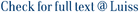We consider an infinite horizon portfolio problem with borrowing constraints, in which an agent receives labor income which adjusts to financial market shocks in a path dependent way. This path dependency is the novelty of the model and leads to an infinite dimensional stochastic optimal control problem. We solve the problem completely and find explicitly the optimal controls in feedback form. This is possible because we are able to find an explicit solution to the associated infinite dimensional HJB equation, even if state constraints are present. To the best of our knowledge, this is the first infinite dimensional generalization of Merton's optimal portfolio problem for which explicit solutions can be found. The explicit solution allows us to study the properties of optimal strategies and discuss their financial implications. © 2020, Society for Industrial and Applied Mathematics

Optimal portfolio choice with path dependent labor income: the infinite horizon case / Biffis, E.; Gozzi, Fausto; Prosdocimi, Cecilia. - In: SIAM JOURNAL ON CONTROL AND OPTIMIZATION. - ISSN 0363-0129. - 58:4(2020), pp. 1906-1938. [10.1137/19M1259687]

### Optimal portfolio choice with path dependent labor income: the infinite horizon case

#### Abstract

We consider an infinite horizon portfolio problem with borrowing constraints, in which an agent receives labor income which adjusts to financial market shocks in a path dependent way. This path dependency is the novelty of the model and leads to an infinite dimensional stochastic optimal control problem. We solve the problem completely and find explicitly the optimal controls in feedback form. This is possible because we are able to find an explicit solution to the associated infinite dimensional HJB equation, even if state constraints are present. To the best of our knowledge, this is the first infinite dimensional generalization of Merton's optimal portfolio problem for which explicit solutions can be found. The explicit solution allows us to study the properties of optimal strategies and discuss their financial implications. © 2020, Society for Industrial and Applied Mathematics
##### Scheda breve Scheda completa Scheda completa (DC)2020
Life-cycle optimal portfolio with labor income; Optimal control problems in infinite dimension in state constraints; Second order Hamilton-Jacobi-Bellman equations in infinite dimension; Stochastic functional (delay) differential equations; Verification theorems and optimal feedback controls; Wages with path dependent dynamics (sticky)
Optimal portfolio choice with path dependent labor income: the infinite horizon case / Biffis, E.; Gozzi, Fausto; Prosdocimi, Cecilia. - In: SIAM JOURNAL ON CONTROL AND OPTIMIZATION. - ISSN 0363-0129. - 58:4(2020), pp. 1906-1938. [10.1137/19M1259687]
File in questo prodotto:
File
BiffisGozziProsdocimiSICON2020.pdf

Solo gestori archivio

Descrizione: Articolo principale
Tipologia: Versione dell'editore
Licenza: Tutti i diritti riservati
Dimensione 558.84 kB
Utilizza questo identificativo per citare o creare un link a questo documento: `https://hdl.handle.net/11385/199535`
•10
•10